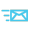•0086-0577-62698923
•sales@huimultd.com
•No.266 Liujiang Road, Wenzhou, Zhejiang, China

location : Home > Terminology Library >

# Apparent Power2019-04-03

Apparent power is the direct product of current and voltage in a circuit. For non-pure resistor circuits, the active power of the circuit is less than the apparent power. For a purely resistive circuit, the apparent power is equal to the active power.

In a sinusoidal AC circuit, the active power is generally less than the apparent power, which means that a discount on the apparent power is equal to the average power. This discount is Cosφ, called the power factor(PF).

The relationship among the apparent power, the active power and the reactive power is: S^2 = P^2 + Q^2

S=Apparent power (VA)

P=Active power (W)

Q=Reactive power (var)